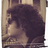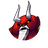Welcome to our new forum
All users of the legacy CODESYS Forums, please create a new account at account.codesys.com. But make sure to use the same E-Mail address as in the old Forum. Then your posts will be matched.

## Sorting an array document.SUBSCRIPTION_OPTIONS = { "thing": "topic", "subscribed": false, "url": "subscribe", "icon": { "css": "fa fa-envelope-o" } };

NBCC
2017-08-22
2021-11-25
<< < 1 2 (Page 2 of 2)
•Ingo - 2020-09-26

You're right. I forgot 😉
A workaround for this can be:

```VAR_INPUT
// reference to first element to sort
array : ANY;
// number of elements in the array
num : DINT;
END_VAR
```

•Hi everyone,

I have taken a look of your examples. In other languages we pass a comparison function as a parameter of the sorting function. This is not (or it is?) possible using ST language.

A way to do it may be to create an ABSTRACT FUNCTION_BLOCK FB_Sort with a private Compare and final Sort method. Then in your code you have to create your own FB_Sort_blablabla (example: FB_Sort_REAL) which derivate (EXTENDS) from the abstract FB_Sort. Then you override the Compare method to fit your needs (like comparing REAL, LREAL, INT, FB_Biscuit, FB_Something, etc…).
You don't have to override the method Sort, it is the same for all your data types.
You don't need to implement anything in the body of your FB_Sort_blablabla.
To sort your array, simply call FB_Sort_blablabla.Sort(insert_all_parameters_as_described).

##### OPEN POINT

Since the function Sort uses an internal array of byte to be able to perform a variable swap, if your data type exceed the length of the array, the Sort method will return FALSE and your array will not be sorted. Maybe we should also pass as parameter the location of the temp variable to ensure that the swap will always be possible?
Or should we also add a method "Swap" which also need to be overriden?
We also can call the operator __NEW() with the needed byte count...

##### Back to the code

See code below (I have attached 2 FB's in PLCopenXML format):

The Abstract function block FB_Sort with abstract method Compare and final method Sort:

```FUNCTION_BLOCK ABSTRACT FB_Sort
(*
* Purpose: Run an optimized version of insertion sort with specified order
* Author: NothinRandom
* v1.0  September 16, 2020
* Note:
*)
VAR_INPUT
END_VAR
```
```// No code in the body, the sort algorithm is done using the Sort method
// We could fill the body of this FB with the code of the Sort method
// but then we cannot prevent someone to override it (or it will cause inatended behaviour)
```

Compare method:

```(* Please override this method so it returns:
* 0 : a == b
*-1 : a < b
* 1 : a > b
* which a and b are the ADDRESS of the 2 elements you want to compare
*)
METHOD ABSTRACT Compare : DINT
VAR_INPUT
a : PVOID;
b : PVOID;
END_VAR
```

```// No code here since it is an abstract method !!!
```

Sort method:

```METHOD FINAL Sort : BOOL
VAR_INPUT
pbBuffer    : POINTER TO BYTE; // pointer to buffer
diElements  : DINT;            // array length
uiDataSize  : UINT;            // use it like this : uiDataType := SIZEOF(MyDataType)
// where MyDataType is the type your currently want to compare
bDescending : BOOL := FALSE;   // default ascending
END_VAR
VAR
_diIndex            : DINT;                 // loop index
_diMinIndex         : DINT;                 // loop lowest index tested
_diMaxIndex         : DINT;                 // loop highest index tested
_diCurrentIndex     : DINT;                 // loop current tested element index
_abTemp             : ARRAY [1..8] OF BYTE; // store temp value
_pbElement1         : PVOID;      // pointer operator, current element
_pbElement2         : PVOID;      // pointer operator, insert element
_pCurrent : PVOID;
END_VAR
```

```IF uiDataSize > 8 THEN
// Not enough space to store the data
Sort := FALSE;
RETURN;
END_IF

FOR _diIndex := 1 TO diElements - 1 DO
// highest index tested
_diMaxIndex := _diIndex;
// lowest index tested
_diMinIndex := -1;
// element we need to insert
_pbElement2 := pbBuffer + TO_UDINT(uiDataSize*_diIndex);

REPEAT
// current test index
_diCurrentIndex := _diMinIndex + (_diMaxIndex-_diMinIndex)/2;
// get element at current test index
_pbElement1 := pbBuffer + TO_UDINT(uiDataSize*_diCurrentIndex);

IF (NOT bDescending) THEN
// IF (_liElement1 > _liElement2) THEN
IF THIS^.Compare(a := _pbElement1, b := _pbElement2) > 0 THEN
_diMaxIndex := _diCurrentIndex;
ELSE
_diMinIndex := _diCurrentIndex;
END_IF
ELSE
IF THIS^.Compare(a := _pbElement1, b := _pbElement2) > 0 THEN
_diMinIndex := _diCurrentIndex;
ELSE
_diMaxIndex := _diCurrentIndex;
END_IF
END_IF

UNTIL
_diMaxIndex - _diMinIndex < 2
END_REPEAT

// Save value to insert
// Move block
n:=uiDataSize*TO_UDINT(_diIndex-_diMaxIndex));
// set key
n:=uiDataSize);
END_FOR

Sort := TRUE;
```

Ok ... that's for the base of all your future sort function block... Let see an example that can be used to sort REAL type:

First create a new FB which EXTENDS from FB_Sort like this (the FINAL keyword is not necessary):

```FUNCTION_BLOCK FINAL FB_Sort_Real EXTENDS FB_Sort
VAR_INPUT
END_VAR
VAR_OUTPUT
END_VAR
VAR
END_VAR
```
```// Leave the body empty
```

Then, simply override the Compare method:

```(* Please override this method so it returns:
* 0 : a == b
*-1 : a < b
* 1 : a > b
* which a and b are the ADDRESS of the 2 elements you want to compare
*)
METHOD  Compare : DINT
VAR_INPUT
a : PVOID;
b : PVOID;
END_VAR
VAR
_a : REAL; //
_b : REAL;
END_VAR
```
```// Here I tried a byte per byte comparison
// Unfortunately this is not working with REAL type... maybe little/big endian issue?
// This could also not work with integer type (INT/UINT, DINT/UDINT, ...)
// Compare := MEMCMP(a, b, SIZEOF(REAL));

// The "cast" method will worked in any case and for any type (like for FB_Something)
IF _a < _b THEN
Compare := -1;
ELSIF _a > _b THEN
Compare := 1;
ELSE
Compare := 0;
END_IF
```

And I use the code like it somewhere inside my project:

```VAR
array_toto : ARRAY [0..9] OF REAL :=
[ 0.5, 0.4,  0.9, 0.2, 0.7, 0.3,0.6, 0.8, 0.1, 1.0];

sort_real : FB_Sort_Real;
END_VAR
---
diElements := 10,
uiDataSize := SIZEOF(REAL),
bDescending := FALSE);
```

👍
1

•dFx - 2021-11-25

I was worried we went a little too far on this topic, but you clearly went a step further.

Just about the real comparaison, real values are stored as IEEE format (see : https://en.wikipedia.org/wiki/Single-precision_floating-point_format#IEEE_754_single-precision_binary_floating-point_format:binary32 ). That's a good exemple why you need to type cast and compare values knowing the datatype you are comparing.

great input 👍

Edit : Typo

👍
1

Last edit: dFx 2021-11-25
•About the MEMCMP/cast in the Compare function: Yes of course a byte per byte comparison is stupid for REAL and also on other types. I was so excited that I forget to remove this commented line of code of my example... shame on me.

We aren't far enough 😅... insertion sort is not that bad but merge sort or quick sort will be really more effective. I am looking to adapt the sort provided in the OSCAT lib to run using a pointer (PVOID) instead of a POINTER TO ARRAY[1..32000] OF REAL. I have some issue with left and right boundaries and/or with the pivot start position. But I will find out soon I hope 😄

My end goal is really to provide a Sort "function" (or class) that can Sort anything using a given compare function. So it will be easy to sort some FB_Biscuit by area size to know which one will get the more chocolate on it 😋

•